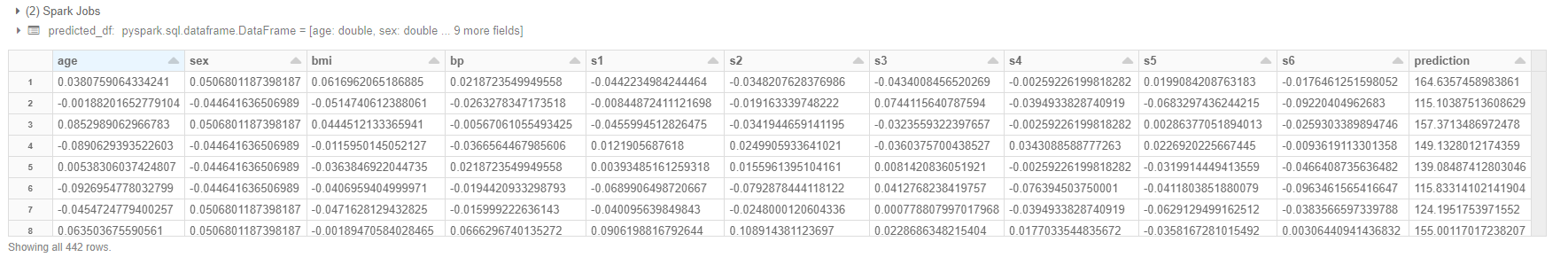Introduction

In the following article, I used Databricks' managed MLflow to train my model and manage my lifecycle.

This time I would like to load the trained and staging model from another notebook. As an image, the trained model is loaded as a Pyspark user-defined function, and the pyspark data frame is distributed.

setup

For the model you want to call ["Run ID"](https://qiita.com/knt078/items/c40c449a512b79c7fd6e#%E3%83%A2%E3%83%87%E3%83%AB%E3%81% Read AE% E7% 99% BB% E9% 8C% B2).

python

# run_id = "<run-id>"
run_id = "d35dff588112486fa1684f38******"
model_uri = "runs:/" + run_id + "/model"

Load the experimented training model using the MLflow API.

python

import mlflow.sklearn
model.coef_

Next, read the diabetes dataset that was also used for training and drop the "progression" column. Then convert the loaded pandas data frame to a pyspark data frame.

python

# Import various libraries including sklearn, mlflow, numpy, pandas

from sklearn import datasets
import numpy as np
import pandas as pd

X = diabetes.data
y = diabetes.target

# Create pandas DataFrame for sklearn ElasticNet linear_model
Y = np.array([y]).transpose()
d = np.concatenate((X, Y), axis=1)
cols = ['age', 'sex', 'bmi', 'bp', 's1', 's2', 's3', 's4', 's5', 's6', 'progression']
data = pd.DataFrame(d, columns=cols)
dataframe = spark.createDataFrame(data.drop(["progression"], axis=1))

Call the MLflow model

Call the trained model as a Pyspark user-defined function using the MLflow API.

python

import mlflow.pyfunc
pyfunc_udf = mlflow.pyfunc.spark_udf(spark, model_uri=model_uri)

Make predictions using user-defined functions.

python

predicted_df = dataframe.withColumn("prediction", pyfunc_udf('age', 'sex', 'bmi', 'bp', 's1', 's2', 's3', 's4', 's5', 's6'))
display(predicted_df)

I was able to do distributed processing using the Pyspark model.in conclusion

This time I was able to call the trained model using the MLflow API and distribute it in Pyspark. Databricks is constantly being updated with new features to make it easier to use. I would like to continue to chase after new features.